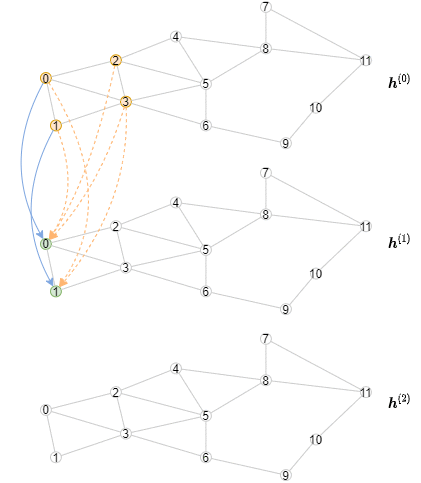# 6.6 超大图上的精准离线推断¶

(English Version)## 实现离线推断¶

class StochasticTwoLayerGCN(nn.Module):
def __init__(self, in_features, hidden_features, out_features):
super().__init__()
self.hidden_features = hidden_features
self.out_features = out_features
self.conv1 = dgl.nn.GraphConv(in_features, hidden_features)
self.conv2 = dgl.nn.GraphConv(hidden_features, out_features)
self.n_layers = 2

def forward(self, blocks, x):
x_dst = x[:blocks.number_of_dst_nodes()]
x = F.relu(self.conv1(blocks, (x, x_dst)))
x_dst = x[:blocks.number_of_dst_nodes()]
x = F.relu(self.conv2(blocks, (x, x_dst)))
return x

def inference(self, g, x, batch_size, device):
"""        用该模块进行离线推断        """
# 逐层计算表示
for l, layer in enumerate([self.conv1, self.conv2]):
y = torch.zeros(g.number_of_nodes(),
self.hidden_features
if l != self.n_layers - 1
else self.out_features)
g, torch.arange(g.number_of_nodes()), sampler,
batch_size=batch_size,
shuffle=True,
drop_last=False)

# 在一层中，依批次对节点进行迭代
for input_nodes, output_nodes, blocks in dataloader:
block = blocks

# 将必要输入节点的特征复制到GPU上
h = x[input_nodes].to(device)

# 计算输出，注意计算方法是一样的，但只对一层进行计算
h_dst = h[:block.number_of_dst_nodes()]
h = F.relu(layer(block, (h, h_dst)))

# 将输出复制回CPU
y[output_nodes] = h.cpu()

x = y

return y